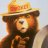# Convert ATR_RSRL indicator to ThinkorSwim#### borntostun

##### New member
Hi all. Here's an indicator that I've used in Tradingview (pinescript) for a while. I'm wondering if it's possible to get this transformed into thinkscript. Thanks!

Code:
``````//@version=2
study(title = "ATR_RSRL", shorttitle = "ATR_RSRL",overlay=true)
length = input(14, minval=1)
src = input(close, title="Source")
mult = input(2.0, minval=0.001, maxval=50)
maLen=input(7,title="maLength")
basis = sma(src, length)
dev = mult * atr(length)
upper = basis + dev
lower = basis - dev
bbr = (src - lower)/(upper - lower)
bbe= ema(bbr,maLen)
up =  bbe>bbe and bbe<bbe?bbe:na
bt =  bbe<bbe and bbe>bbe?bbe:na

topH=na(up)==0?highest(3):na
bottomL=na(bt)==0?lowest(3):na

tf= fixnan(topH)
bf =fixnan(bottomL)

btop=close>open?close:open
bbot=close>open?open:close

plot(tf,color=red,style=circles,linewidth=1,offset=-1)
plot(bf,color=green,style=circles,linewidth=1,offset=-1)``````

Last edited by a moderator:
D

#### diazlaz

##### Well-known member
2019 Donor
VIP
thanks for your suggestion, very nice - it's simple and effective. here you go @borntostun. please share how you use it and if anyone has any good enhancements please share it back with the community.

OriginalPortCode:

Ruby:
``````#ATR_RSRL indicator to ThinkorSwim
#
#CREDITS
# tongue1
#
#CHANGELOG
# 2019.12.10 1.0 @diazlaz - Initial Port/Interperation
#
#REQUEST
# @borntostun
#
#

declare upper;

#INPUTS
input length = 14;
input mult = 2.0;
input maLen = 7;
input showLabels = yes;

#LOGIC
def src = close;
def basis = Average(src, length);
def dev = mult * atr(length);
def upper = basis + dev;
def lower = basis - dev;
def bbr = (src - lower)/(upper - lower);
def bbe = expAverage(bbr, maLen);

def na = Double.NaN;
def up =  if bbe > bbe and bbe < bbe then bbe else 0;
def bt =  if bbe < bbe and bbe > bbe then bbe else 0;

def topH = if up != 0 then highest(high, 3) else topH;
def bottomL = if bt != 0 then lowest(low, 3) else bottomL;

def btop = if close > open then close else open;
def bbot = if close > open then open else close;

#PLOTS
plot pTF = topH;
pTF.SetPaintingStrategy(paintingStrategy = PaintingStrategy.DASHES);
pTF.SetDefaultColor(COLOR.RED);

plot pBF = bottomL;
pBF.SetPaintingStrategy(paintingStrategy = PaintingStrategy.DASHES);
pBF.SetDefaultColor(COLOR.GREEN);

#LABELSHow do I convert this ATR% chart study into a scanner watchlist column? Questions 5